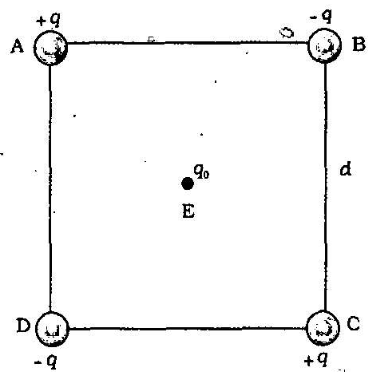Home/Class 12/Physics/

## QuestionPhysicsClass 12

Four charges are arranged at the corners of a square  $$\mathit{ABCD}$$  of side$$d$$. as shown in the figure.  $$A$$  charge  $$q_0$$  is brought to the centre  $$E$$  of the square, the four charges being held fixed at its corners. How much extra work is needed to do this?The extra work necessary to bring a charge $$q_{0}$$ to the point E when the four charges are at $$A,\;B\;,\;C$$ and $$D$$ is  given by
$$W\;=\;{q}_{0}\;\times \;V$$
$$\Rightarrow$$$${q}_{0}\;$$$$\times$$ electrostatic potential at  $$E$$  due to the charges at  $$A,\;B\;,C$$ and $$D$$) The electrostatic potentlal at
is clearly zero since potential due to  $$\text A$$  and  $$\text C$$is cancelled by that due to  $$\text B$$  and  $$\text D$$  Hence. no work is. required to bring any charge to point  $$\mathit{E.}$$